# Lens Calculations using Refractive Index

• nao113
In summary, the author discusses how to find Δ using h, r, and d. However, they make an error in their calculations and suggest using the sagitta theorem, which the author learned in Jr High School.f

#### nao113

Homework Statement
Consider a lens made of glass with refractive index 𝑛𝑛=1.5 is placed in the air (refractive index =1.0), as shown in the figure. The first surface is plane and the second surface is spherical convex shape whose radius of curvature is 𝑟𝑟=100 [mm]. z-axis is called optical axis. The first surface perpendicularly intersects the optical axis at O, and second surface intersects the optical axis at Q. The thickness of the lens OQ = 𝑑𝑑 = 20 [mm]. A light beam parallel to the optical axis is incident to the lens, where the distance (height) of the beam from the optical axis is h. The refracted beam intersects the optical axis at X. Derive the distance between the lens and the intersection X, Q
Relevant Equations
n1theta1 = n2 theta2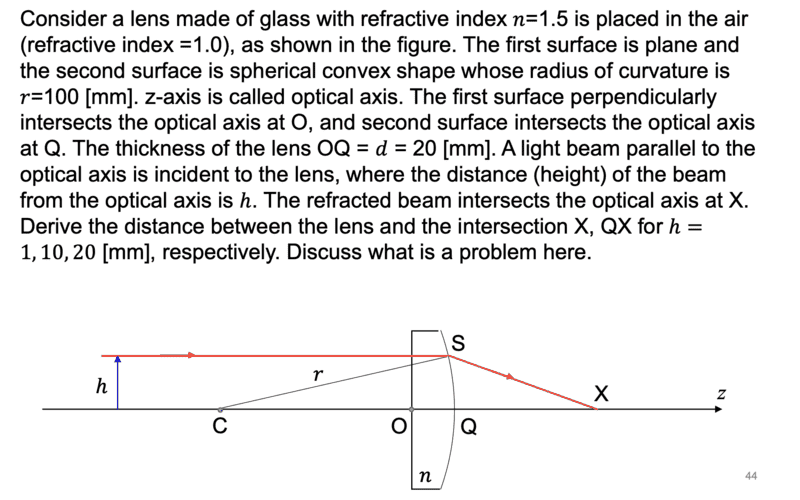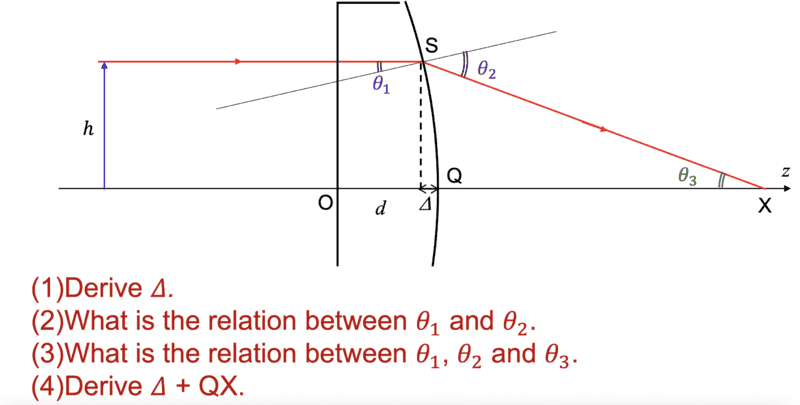I already found the answer for Sin theta 2 like the pic below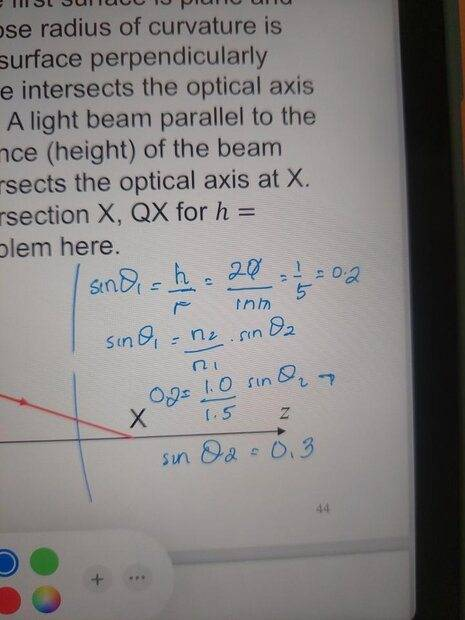But, I am still not sure about how to derive delta here. Can anyone show me they way? thank you

Can you find ##\theta_3## in terms of ##\theta_1## and ##\theta_2##? Then use Snell's law to eliminate ##\theta_2##.

Can you find ##\theta_3## in terms of ##\theta_1## and ##\theta_2##? Then use Snell's law to eliminate ##\theta_2##.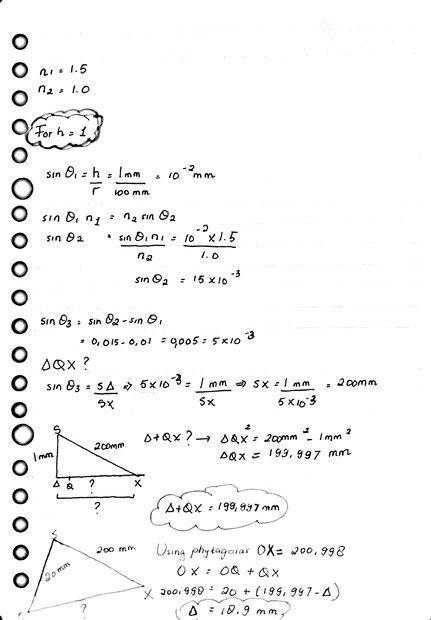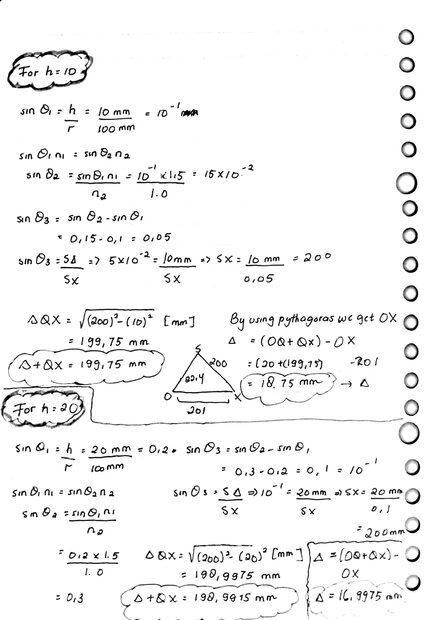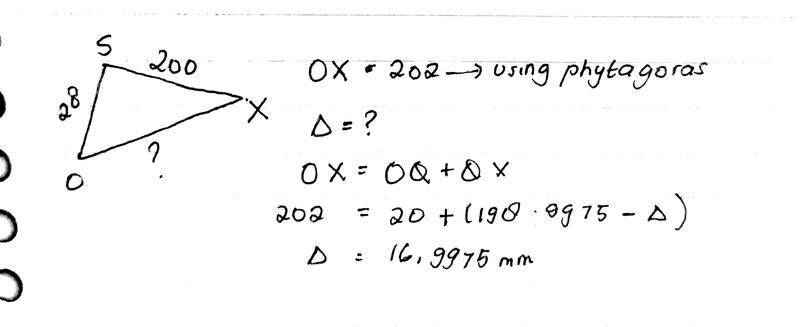I put it like this, how is it?

It doesn't look good. How about finding a formula for Δ in terms of ##h##, ##r## and ##d##? Then instead of doing three separate calculations, you can substitute three separate values in the one formula. Even better, load the formula on a spreadsheet. That way it is less likely that you will get an inconsistent answer, you and we can troubleshoot your work more easily.

I see one glaring error that makes your numerical answers incorrect. That is your assertion that $$\sin\theta_3=\sin\theta_2-\sin\theta_1.~~\leftarrow~~\text{This is not correct}$$Why do you think that the sines are related this way? The way to do this problem is to answer the four questions in the order (2), (3), (4) and (1). So let's start with (2), "What is the relation between ##\theta_1##, ##\theta_2## and ##\theta_3##?"

•Bystander and berkeman
How about finding a formula for Δ in terms of h, r and d?
Hint: This relationship is a standard piece of lens/mirror plane geometry known as the sagitta theorem. Learn it.

•berkeman
Hint: This relationship is a standard piece of lens/mirror plane geometry known as the sagitta theorem. Learn it.
I didn't know it had a theorem name. I always (re)derived it as needed using the Pythagorean theorem.

I remember it because I ground a telescope mirror in Jr High school.
Now I am lucky to remember to put on pants...

••kuruman and berkeman